# GMAT Math : Calculating the surface area of a cylinder

## Example Questions

### Example Question #1 : Calculating The Surface Area Of A Cylinder

What is the surface area of a cylinder with a radius of 7 and a height of 3?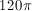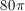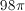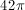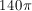Explanation:

All we really need here is to remember the formula for the surface area of a cylinder.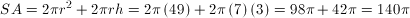### Example Question #2 : Calculating The Surface Area Of A Cylinder

The height of a cylinder is twice the circumference of its base. The radius of the base is 9 inches. What is the surface area of the cylinder?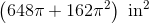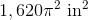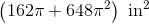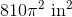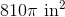Explanation:

The radius of the base is 9 inches, so its circumference istimes this, or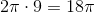inches. The height is twice this, orinches.

Substitute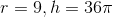in the formula for the surface area of the cylinder: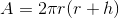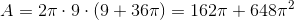square inches

### Example Question #3 : Calculating The Surface Area Of A Cylinder

Calculate the surface area of the following cylinder.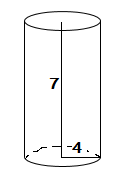(Not drawn to scale.)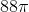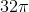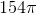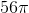Explanation:

The equation for the surface area of a cylinder is: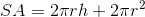we plug in our values: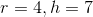to find the surface area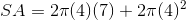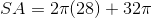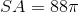### Example Question #4 : Calculating The Surface Area Of A Cylinder

Calculate the surface area of the following cylinder.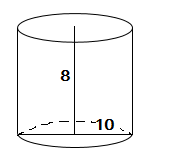(Not drawn to scale.)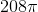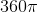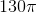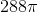Explanation:

The equation for the surface area of a cylinder isWe plug in our values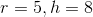into the equation to find our answer.

Note: we were given the diameter of the cylinder (10), in order to find the radius we had to divide the diameter by two.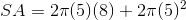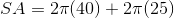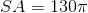### Example Question #1 : Cylinders

A cylinder has a height of 9 and a radius of 4. What is the total surface area of the cylinder?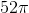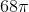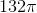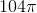Explanation:

We are given the height and the radius of the cylinder, which is all we need to calculate its surface area. The total surface area will be the area of the two circles on the bottom and top of the cylinder, added to the surface area of the shaft. If we imagine unfolding the shaft of the cylinder, we can see we will have a rectangle whose height is the same as that of the cylinder and whose width is the circumference of the cylinder. This means our formula for the total surface area of the cylinder will be the following: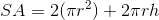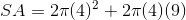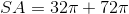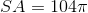### Example Question #6 : Calculating The Surface Area Of A Cylinder

Grant is making a canister out of sheet metal. The canister will be a right cylinder with a height ofmm. The base of the cylinder will have a radius of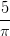mm. If the canister will have an open top, how many square millimeters of metal does Grant need?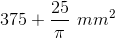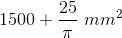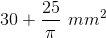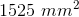Explanation:

This question is looking for the surface area of a cylinder with only 1 base. Our surface area of a cylinder is given by: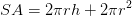However, because we only need 1 base, we can change it to: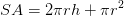We know our radius and height, so simply plug them in and simplify.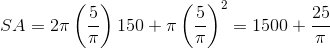### Example Question #2 : Calculating The Surface Area Of A Cylinder

Find the surface area of a cylinder whose height isand radius is.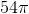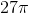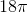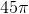Explanation:

To find the surface area of a cylinder, you must use the following equation.Thus,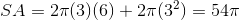### Example Question #8 : Calculating The Surface Area Of A Cylinder

A right circular cylinder has bases of radius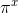; its height is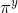. Give its surface area.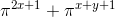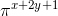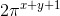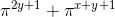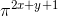Explanation:

The surface area of a cylinder can be calculated from its radius and height as follows: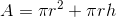Setting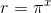and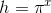: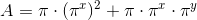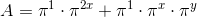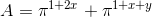or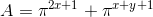Tired of practice problems?

Try live online GMAT Math prep today.